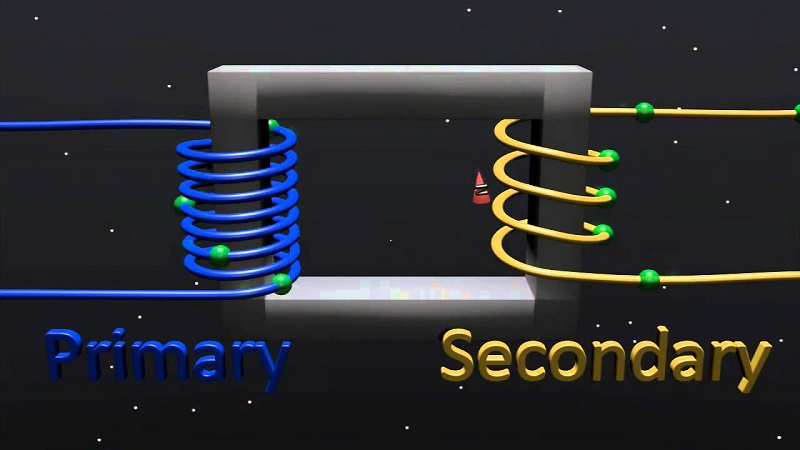# Circuit Theory - EE8251

## Online Study Material, Lecturing Notes, Assignment, Reference, Wiki and important questions and answers## UNIT I BASIC CIRCUITS ANALYSIS

=> Fundamentals of Communication Engineering
=> Basic Circuits Analysis
=> Important Short Questions and Answers: Basic Circuits Analysis
=> Basic Elements and Introductory Concepts
=> Kirchoff's Law with Example Problems and Calculations
=> DC Circuits and AC Circuits
=> Difference Between AC And DC
=> Problem on Parallel Networks
=> Mesh Analysis
=> Nodal Analysis

## UNIT II NETWORK REDUCTION AND THEOREMS FOR DC AND AC IRCUITS

=> Network Reduction and Network Theorems for DC And AC Circuits
=> Important Short Questions and Answers: Network Reduction and Network Theorems for DC And AC Circuits
=> Potential Divider
=> Star Delta Conversion
=> Thevenins Theorem
=> Norton’s Theorem

## UNIT III TRANSIENT RESPONSE ANALYSIS

=> Transient Response For DC Circuits
=> Current through purely resistive circuit, inductance and capacitance
=> Current lags and leads voltage in R-L series circuit
=> Phasor diagram for a series RLC circuit
=> Solved Problems: Transient Response For DC Circuits
=> Time constant and step response of series RL circuit
=> Time constant and step response of series RC circuit
=> Analysis of undriven(source free) series RC and RL circuits
=> Transient Response of RL Circuits
=> Transient Response of RC Circuits
=> Transient Response of RLC Circuits
=> Characterization of Two Port Networks in Terms Of Z,Y and H Parameters
=> Important Short Questions and Answers: Transient Response For DC Circuits

## UNIT IV THREE PHASE CIRCUITS

=> Analysing Three Phase Circuits
=> Important Short Questions and Answers: Analysing Three Phase Circuits
=> Star connection and Star connection
=> Solved Problems: Analysing Three Phase Circuits

## UNIT V RESONANCE AND COUPLED CIRCUITS

=> Resonance and Coupled Circuits
=> The step response of series RLC circuit
=> Expressions for Q-factor of inductor and capacitor
=> linear transformer
=> Solved Problems: Resonance and Coupled Circuits
=> Important Short Questions and Answers: Resonance and Coupled Circuits

EE6201 Circuit Theory - Anna University 2013 Regulation Syllabus - Download Pdf
EE8251 Circuit Theory - Anna University 2017 Regulation Syllabus - Download Pdf
098 - EE8251, EE6201 Circuit Theory - Important Questions - Download Pdf

098 - EE8251, EE6201 Circuit Theory - EE2151 Circuit Theory May June 2013 Regulation 2008 Question Paper
098 - EE8251, EE6201 Circuit Theory - EE2151 Circuit Theory May June 2014 Regulation 2008 Question Paper
098 - EE8251, EE6201 Circuit Theory - EE2151 Circuit Theory May June 2015 Regulation 2008 Question Paper
098 - EE8251, EE6201 Circuit Theory - EE2151 Circuit Theory Nov Dec 2012 Regulation 2008 Question Paper
098 - EE8251, EE6201 Circuit Theory - EE2151 Circuit Theory Nov Dec 2014 Regulation 2008 Question Paper
098 - EE8251, EE6201 Circuit Theory - EE6201 Circuit Theory May June 2014 Regulation 2013 Question Paper
098 - EE8251, EE6201 Circuit Theory - EE6201 Circuit Theory May June 2015 Regulation 2013 Question Paper
098 - EE8251, EE6201 Circuit Theory - EE6201 Circuit Theory May June 2016 Regulation 2013 Question Paper
098 - EE8251, EE6201 Circuit Theory - EE6201 Circuit Theory Nov Dec 2014 Regulation 2013 Question Paper
098 - EE8251, EE6201 Circuit Theory - EE6201 Circuit Theory Nov Dec 2015 Regulation 2013 Question Paper